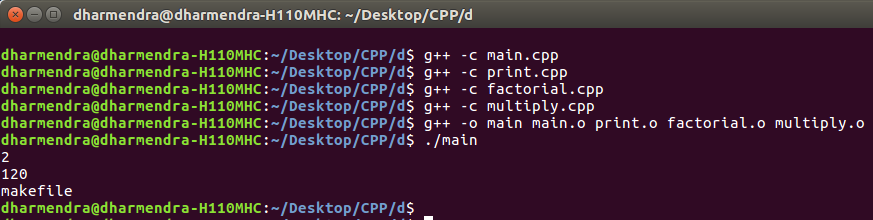Related Articles
MakeFile in C++ and its applications
• Difficulty Level : Easy
• Last Updated : 02 Nov, 2018

Given a C++ program and the task is to break the entire program in the form of Makefile.
It is basically used to create .cpp file and .h file for each class/functions and its functionalities and later link them all through a Makefile command available in C++.

Advantages:

• It makes codes more concise and clear to read and debug.
• No need to compile entire program every time whenever you make a change to a functionality or a class. Makefile will automatically compile only those files where change has occurred.
• Generally, in long codes or projects, Makefile is widely used in order to present project in more systematic and efficient way.

Example: Create a program to find the factorial and multiplication of numbers and print it.

Traditional Way

 `// Program to calculate factorial and``// multiplication of two numbers.``#include``using` `namespace` `std;`` ` `// Function to find factorial``int` `factorial(``int` `n)``{``    ``if` `(n == 1)``        ``return` `1;``    ``// Recursive Function to find ``    ``// factorial``    ``return` `n * factorial(n - 1);``}`` ` `// Function to multiply two numbers``int` `multiply(``int` `a, ``int` `b)``{``    ``return` `a * b;``}`` ` `// Function to print``void` `print()``{``    ``cout << ``"makefile"` `<< endl;``}`` ` `// Driver code``int` `main()``{``    ``int` `a = 1;``    ``int` `b = 2;``    ``cout << multiply(a, b) << endl;``    ``int` `fact = 5;``    ``cout << factorial(5) << endl;``    ``print();``    ``return` `0;``}`

Output:

```2
120
makefile```

Use Makefile to run the above program:

• File Name: main.cpp
 `#include `` ` `// Note function.h which has all functions``// definations has been included``#include "function.h"`` ` `using` `namespace` `std;`` ` `// Main program``int` `main()``{``    ``int` `num1 = 1;``    ``int` `num2 = 2;``    ``cout << multiply(num1, num2) << endl;``    ``int` `num3 = 5;``    ``cout << factorial(num3) << endl;``    ``print();``}`
• File Name: print.cpp
 `#include `` ` `// Definition of print function is``// present in function.h file``#include "function.h"``using` `namespace` `std;`` ` `void` `print()``{``    ``cout < ``"makefile"` `<< endl;``}`
• File Name: factorial.cpp
 `#include `` ` `// Definition of factorial function``// is present in function.h file``#include "function.h"``using` `namespace` `std;`` ` `// Recursive factorial program``int` `factorial(``int` `n)``{``    ``if` `(n == 1)``        ``return` `1;``    ``return` `n * factorial(n - 1);``}`
• File Name: multiply.cpp
 `#include `` ` `// Definition of multiply function ``// is present in function.h file``#include "function.h"``using` `namespace` `std;`` ` `int` `multiply(``int` `a, ``int` `b)``{``    ``return` `a * b;``}`
• File Name: functions.h
 `#ifndef FUNCTIONS_H``#define FUNCTIONS_H`` ` `void` `print();``int` `factorial(``int``);``int` `multiply(``int``, ``int``);`` ` `#endif`

Commands to Compile and Run above program:

```Open Terminal and type commands:
g++ -c main.cpp
g++ -c print.cpp
g++ -c factorial.cpp
g++ -c multiply.cpp
g++ -o main main.o print.o factorial.o multiply.o
./main

Note: g++ -c filename.cpp is used to create object file.
```Want to learn from the best curated videos and practice problems, check out the C++ Foundation Course for Basic to Advanced C++ and C++ STL Course for foundation plus STL.  To complete your preparation from learning a language to DS Algo and many more,  please refer Complete Interview Preparation Course.
My Personal Notes arrow_drop_up